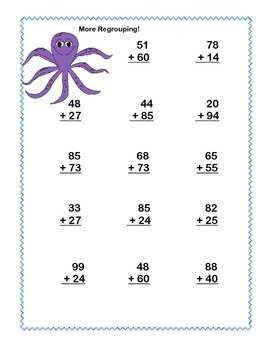# Second Grade Math Review Bundle-Ocean Theme 4 CCSSSubject
Resource Type
File Type

PDF

(7 MB|61 pages)
Standards
• Product Description
• StandardsNEW
This is a fun and complete way to review second grade math skills. It includes a 32 page packet and 2 sets of task cards for a total of 61 pages all with a whimsical charming ocean theme.
It includes 3 products that are offered in my store separately. There is a 20% reduction in cost if purchased in this bundle.
The bundle includes:
32 page packet with an Under the Sea Theme
14 practice pages and an Answer Key
These sequenced worksheets can be used as morning work or with any math program as introduction or practice.
Included are worksheets on the following:
Comparing Numbers using the
greater and less symbols
Adding a 2 digit and 1 digit numbers in columns
Adding two 2 digit numbers in columns no regrouping
Adding three 2 digit numbers in columns
Subtracting a 2 digit number from a 2 digit number no regrouping
Subtracting a 2 digit number from a 2 digit number with regrouping
Subtracting a 3 digit number from a 3 digit number no regrouping
Aligned with
CCSS.Math.Content.2.NBT.A.4
CCSS.Math.Content.2.NBT.B.5
CCSS.Math.Content.2.NBT.B.6

two sets of task cards with 24 cards in each set
Identifying even and odd numbers.
There is a student answer sheet for each set and an answer key for each.
Aligned with:
CCSS.Math.Content.2.OA.C.3

Adding Three and Four Two-digit Numbers Ocean Theme
This collection has 32 task cards to practice adding three and four
two-digit numbers.
A student answer sheet and key are included.

Determine whether a group of objects (up to 20) has an odd or even number of members, e.g., by pairing objects or counting them by 2s; write an equation to express an even number as a sum of two equal addends.
Add up to four two-digit numbers using strategies based on place value and properties of operations.
Fluently add and subtract within 100 using strategies based on place value, properties of operations, and/or the relationship between addition and subtraction.
Compare two three-digit numbers based on meanings of the hundreds, tens, and ones digits, using >, =, and < symbols to record the results of comparisons.
Total Pages
61 pages
Included
Teaching Duration
Other
Report this Resource to TpT
Reported resources will be reviewed by our team. Report this resource to let us know if this resource violates TpT’s content guidelines.